# Increasing Current in Coil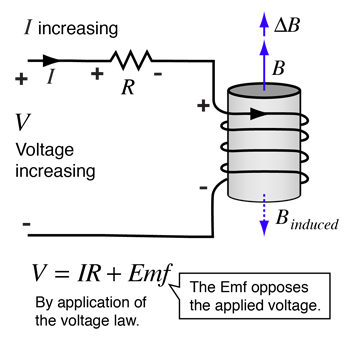Increasing current in a coil of wire will generate a counter emf which opposes the current. Applying the voltage law allows us to see the effect of this emf on the circuit equation. The fact that the emf always opposes the change in current is an example of Lenz's law.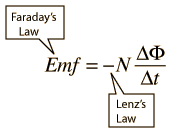The relation of this counter emf to the current is the origin of the concept of inductance. The inductance of a coil follows from Faraday's law.
 Transient behavior of coil
Index

Inductance concepts

 HyperPhysics***** Electricity and Magnetism R Nave
Go Back

# Inductance of a CoilCoil's reaction to increasing current

For a fixed area and changing current, Faraday's law becomes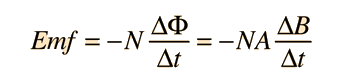Since the magnetic field of a solenoid is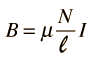then for a long coil the emf is approximated by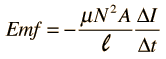From the definition of inductance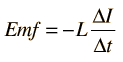we obtain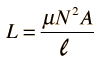Inductance of a solenoid
Index

Inductance concepts

AC Circuits

 HyperPhysics***** Electricity and Magnetism R Nave
Go Back